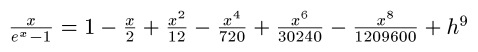## Saturday, November 10, 2012

### Bernoulli Numbers and Polynomials

Today is a good day. I went to the library of alma mater, Cal Poly Pomona for library day. Once a month I try to visit a university library and peruse through with Mathematics Section.I finally got the concept of generating functions. In the many years I studied math, generating functions proved to be an elusive topic for me. Not any more.

What are generating functions?

Generating Functions

Generating functions is a power series. The series is usually does not terminate. The coefficients of the power series can reveal a sequence used in various fields in science.

The Ordinary Generating Function:

G(a_n; x^n) = ∑ a_n * x^n

Sometimes we have an Exponential Generating Function:

E(a_n; x^n) = ∑ a_n * (x^n/n!)

There are other types of generating functions, but I will focus on the basic types described above.

n! represents the factorial of n. In this case, n is a positive integer, and:

n! = n * (n-1) * (n-2) * ... * 3 * 2 * 1

By definition, 0! = 1

To expand the generating function G(a_n; x^n), calculate a Maclaurin Series of G(x). A Maclaurin Series of a function is a Taylor Series about the point x = 0. Hence the Maclaurin Series:

G(x) = G(0) + G'(0) * x/1! + G''(0) * x^2/2! + G'''(0) * x^3/3! + .... + O(x)

where O(x) is the error term G^(n+1)(z) * x^(n+1)/(n+1)! In practice and calculation, O(x) is sometimes "ignored".

An Example: A Basic Generating Function

Expand the generating function, let's go five terms:

G(a_n; x^n) = 1/(1 - x)

G(x) = 1/(1 - x)
Then:
G(0) = 1/(1 - 0) = 1

dG/dx = 1/(x-1)^2; dG/dx(0) = 1

d^2G/dx^2 = -2/(x-1)^3; d^2G/dx^2(0) = 2

d^3G/dx^3 = 6/(x-1)^4; d^3G/dx^3(0) = 6

d^4G/dx^4 = -24/(x-1)^5; d^4G/dx^4(0) = 24

To five terms...

1/(1-x) = 1 + 1 * x/1! + 2 * x^2/2! + 6 *x^3/3! + 24 * x^4/4! + O(x)
= 1 + x + x^2 + x^3 + x^4 + O(x)

The sequence of coefficients are: {1, 1, 1, 1, 1}.

The Bernoulli Numbers

The Bernoulli Numbers can be found by using the Exponential Generating Function:

E(a_n; x) = ∑ x/(e^x - 1)

The Bernoulli Numbers, B_n, are the "coefficients" of the expansion of ∑ x/(e^x - 1). Recall in the Exponential Generating Function, the "coefficients" are of the term x^n/n!.

With E(x) = x/(e^x - 1), the first two derivatives are:

dE/dx = - ((x-1)*e^x + 1)/(e^(2x) - 2*e^x + 1)

d^2E/dx^2 =
((x - 2)*e^(2x) + (x + 2)*e^x) / (e^(3x) - 3*e^(2x) + 3*e^(2x) - 1)

Note that calculating E(0) gives us 0/0. Same for dE/dx(0) and d^2E/dx^2(0). However, if I use a calculate with CAS capabilities such as the Hewlett Packard HP 50g, or mathematical software such as MathStudio (an app for smartphones and iPads), I get something like this (first eight terms):Why is that?

Observe that:

lim x/(e^x - 1) as x → 0 = 0/0

Using the L'Hospital's rule, we can take the derivatives of both numerator and denominator,

lim 1/(e^x) as x → 1 = 1

which implies that:

lim x/(e^x - 1) as x → 0 = 1

You can generate terms by taking the limit as x → 0 for each term.

This how we end up with the series. Now to extract the "coefficients", observe that:

12 = 6 * 2!
(no term contains x^3/3!)
720 = 30 * 4!
(no term contains x^5/5!)
30240 = 42 * 6!
(no term contains x^7/7!)
1,209,600 = 30 * 8!

Our sequence for this generating function (for nine terms) is:

{1, -1/2, 1/6, 0, -1/30, 0, 1/42, 0, -1/30}

These numbers are the Bernoulli numbers. In fact, definition by generating function is:

x/(e^x - 1) = ∑ B_n * (x^n/n!)

Bernoulli Numbers

B_0 = 1
B_1 = -1
B_2 = 1/6
B_3 = 0
B_4 = -1/30
B_6 = 1/42
B_8 = -1/30
B_10 = 5/66
B_12 = -691/2730
B_14 = 7/6

B_n = 0 where n is odd and n > 2

Bernoulli Polynomials

Bernoulli Polynomials can be generated by the following formula:

β_n(x) = ∑((B_k * n!/(k!*(n-k)!) * x^k, from k = 0 to n)

where B_k is the kth Bernoulli number.

Source:
Krylov, Vladimir Ivanoch, translated by H. Stroud Approximate Calculations of Integrals McMillian Company: New York, 1962

Until next time, be safe everyone! Eddie

This blog is property of Edward Shore, 2012.

#### 1 comment:

1.Thanks for sharing stuff related to bernoulli's numbers and polynomials. Quite informative. I was searching information about basic calculator
but your stuff is very useful .Good work .

### Σ(1 / (a^n)) from n=1 to m

Σ(1 / (a^n)) from n=1 to m This blog entry covers the sum of the series: Σ[1 / (a^n), n=1 to m] with n and m positive integers Specific Cas...